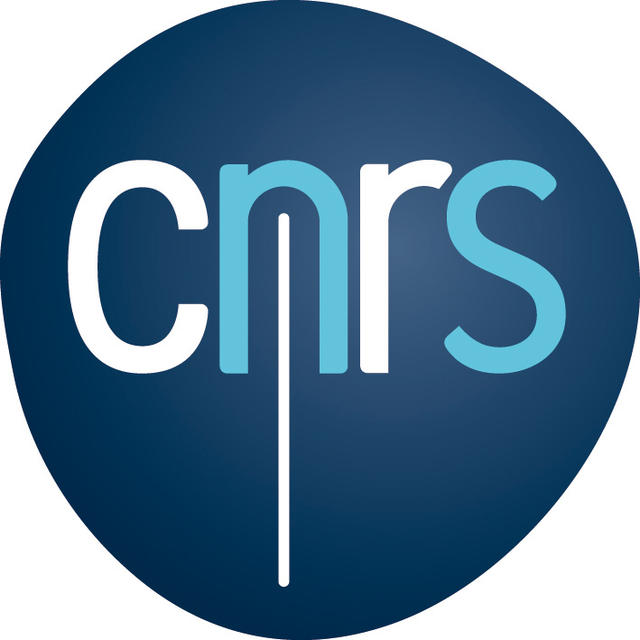List of Participants Program Travel Information Hotel Organizers: Institut Camille Jordan Université Claude Bernard Lyon 1 Contact: slc (at) math.univ-lyon1.fr SLC home page80th Séminaire Lotharingien de Combinatoire March 25-28, 2018 Institut Camille Jordan - Bâtiment Braconnier Université Claude Bernard Lyon 1 43, Bd du 11 novembre 1918 69622 Villeurbanne - FranceThe invited speakers of this session are: Boris Adamczewski (CNRS - Université Claude Bernard Lyon 1, France) Diagonals of rational functions Abstract. A very rich interplay between combinatorics, arithmetic, and geometry arises in the study of homogeneous linear differential equations and especially of those that “come from geometry” and the related study of Siegel G-functions.  A remarkable result is that, by adding variables, analytic solutions of many such differential equations arise in a natural way from much more elementary functions: multivariate rational functions. The underlying process is called diagonalization. More generally, it allows one to associate with any multivariate power series with coefficients in any ring a one-variable power series (by collecting only the coefficients on its diagonal). Applying this operation to multivariate rational power series leads to the class of diagonals of rational functions, or just diagonals for short. The aim of these lectures is to provide an introduction to diagonals. After describing their main properties, I will discuss some arithmetical problems involving their reduction mod p and their link with automata theory. Stéphane Gaussent (Université Jean Monnet Saint-Étienne, France) On some Iwahori-Hecke algebras associated to Kac-Moody groups Abstract. In the first introductory lecture, we will recall some defintions of various Iwahori-Hecke algebras. Then, we will end the talk with the computation of the structure constants of the spherical Hecke algebras in terms of Hecke paths. In the second talk, we will explain how to generalise some algebras defined earlier to the context of Kac-Moody root systems. In the last talk, we will explain how to prove a Macdonald's formula in the Kac-Moody setting. The formula we talk about here is the one giving the image of the Satake isomorphism between the spherical Hecke algebra and the algebra of (generalize) symmetric functions.